# How to plot data from a text file using Matplotlib?

• Last Updated : 13 Jan, 2022

Perquisites: Matplotlib, NumPy

In this article, we will see how to load data files for Matplotlib. Matplotlib is a 2D Python library used for Date Visualization. We can plot different types of graphs using the same data like:

• Bar Graph
• Line Graph
• Scatter Graph
• Histogram Graph and many.

In this article, we will learn how we can load data from a file to make a graph using the “Matplotlib” python module. Here we will also discuss two different ways to extract data from a file.  In the First Module, we will discuss extracting data using the inbuild CVS module and In the Second Module, we will use a third-party “NumPy” Module to extract data from a file.

Requirement:

A text file from where data should be extracted.  Let the file name = GFG.txt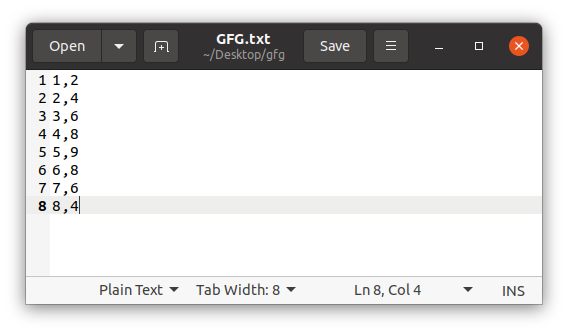Method 1: In this method, we will extract data using CSV module to load CVS files.

Step 1:

Import all required modules.

## Python3

 `import` `matplotlib.pyplot as plt``import` `csv`

Step 2: Create X and Y variables to store X-axis data and Y-axis data from a text file.

## Python3

 `import` `matplotlib.pyplot as plt``import` `csv` `X ``=` `[]``Y ``=` `[]`

Step 3: Open text file in read mode. Pass ‘file_name’ and delimiter in reader function and store returned data in a new variable.

## Python3

 `import` `matplotlib.pyplot as plt``import` `csv` `X ``=` `[]``Y ``=` `[]` `with ``open``(``'GFG.txt'``, ``'r'``) as datafile:``    ``plotting ``=` `csv.reader(datafile, delimiter``=``','``)`

Step 4: Create a loop, that will append the data in X and Y variable.

## Python3

 `import` `matplotlib.pyplot as plt``import` `csv` `X ``=` `[]``Y ``=` `[]` `with ``open``(``'GFG.txt'``, ``'r'``) as datafile:``    ``plotting ``=` `csv.reader(datafile, delimiter``=``','``)``    ` `    ``for` `ROWS ``in` `plotting:``        ``X.append(``int``(ROWS[``0``]))``        ``Y.append(``int``(ROWS[``1``]))`

Step 5: Now pass all the parameter in their respective functions.

## Python3

 `import` `matplotlib.pyplot as plt``import` `csv` `X ``=` `[]``Y ``=` `[]` `with ``open``(``'GFG.txt'``, ``'r'``) as datafile:``    ``plotting ``=` `csv.reader(datafile, delimiter``=``','``)``    ` `    ``for` `ROWS ``in` `plotting:``        ``X.append(``int``(ROWS[``0``]))``        ``Y.append(``int``(ROWS[``1``]))` `plt.plot(X, Y)``plt.title(``'Line Graph using CSV'``)``plt.xlabel(``'X'``)``plt.ylabel(``'Y'``)``plt.show()`

Output: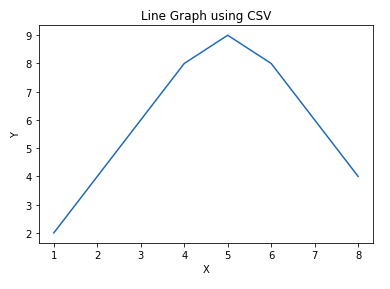Method 2: In this method, we will extract data using numpy module to load files. Here you will notice that Step 2,3 and 4 are replaced by np.loadtxt( )

## Python3

 `import` `matplotlib.pyplot as plt``import` `numpy as np` `X, Y ``=` `np.loadtxt(``'GFG.txt'``, delimiter``=``','``, unpack``=``True``)` `plt.bar(X, Y)``plt.title(``'Line Graph using NUMPY'``)``plt.xlabel(``'X'``)``plt.ylabel(``'Y'``)``plt.show()`

Output: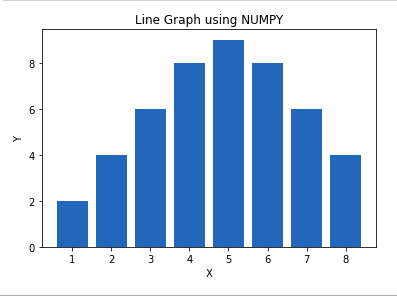You can also try other different graphs by just changing 1 line

plt.plot(X,Y) to plt.scatter(X,Y) or plt.plot(X,Y)

## Python3

 `import` `matplotlib.pyplot as plt``import` `numpy as np` `X, Y ``=` `np.loadtxt(``'GFG.txt'``, delimiter``=``','``, unpack``=``True``)` `plt.plot(X, Y)``plt.title(``'Line Graph using NUMPY'``)``plt.xlabel(``'X'``)``plt.ylabel(``'Y'``)``plt.show()`

Output: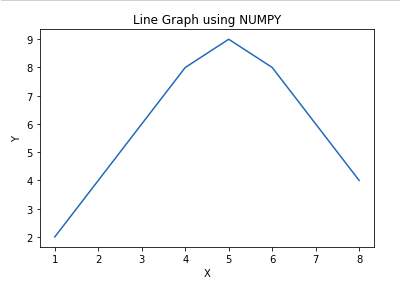## Python3

 `import` `matplotlib.pyplot as plt``import` `numpy as np` `X, Y ``=` `np.loadtxt(``'GFG.txt'``, delimiter``=``','``, unpack``=``True``)` `plt.scatter(X, Y)``plt.title(``'Line Graph using NUMPY'``)``plt.xlabel(``'X'``)``plt.ylabel(``'Y'``)``plt.show()`

Output: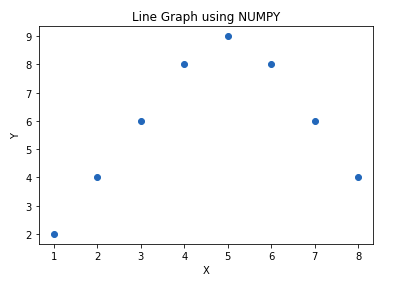My Personal Notes arrow_drop_up